Categories

[h5p id="14"]

# Understand the problem

Let$a_n = n+\frac{1}{n} , n \in \mathbb{N}$. Then the sum of the series$\sum_{n=1}^{\infty} (-1)^{n+1} \frac{a_{n+1}}{n!} is$ (A)$e^{-1}-1$ (B)$e^{-1}$ (C)$1-e^{-1}$ (D)$1+e^{-1}$
##### Source of the problem
IIT JAM 2018 Problem 13
Series
Easy
##### Suggested Book
Real Analysis By S.K Mapa

Do you really need a hint? Try it first!

Consider$a_n = n + \frac{1}{n} , n \in \mathbb{N}$ We have to use$e^{x} = 1 + \frac{x}{1!} + \frac{x^{2}}{2!}+.....$ Specifically$e^{1} = 1+ \frac{1}{1!} + \frac{1}{2!}+....$ And$e^{-1} = 1 - \frac{1}{1!} + \frac{1}{2!} - \frac{1}{3!} + .......$ Do you want to play with it$\sum_{n=1}^{\infty} (-1)^{n+1} \frac{a_{n+1}}{n!} = \sum_{n=1}^{\infty} (-1)^{n+1} \frac{n+1 + \frac{1}{n+1}}{n!}$ =$\sum_{n=1}^{\infty}[ (-1)^{n+1} \frac{1}{(n-1)!} + (-1)^{n+1} \frac{1}{n!} + (-1)^{n+1} \frac{1}{(n+1)!}]$   Now we will be breaking it term by term for the ease of calculation. Can you do it from here?$\sum_{n=1}^{\infty} (-1)^{n+1} \frac{a_{n+1}}{n!}$ =$[1-\frac{1}{1!} + \frac{1}{2!} - ......] + [\frac{1}{1!} - \frac{1}{2!} + \frac{1}{3!} - .....] + [\frac{1}{2!} - \frac{1}{3!} + \frac{1}{4!} -.......]$ =$e^{-1} + [1-e^{-1}] + [e^{-1} + 1 - 1]$ =$e^{-1} + 1$ So option (D) is our required answer.

# The will look more easy if we take a look into the knowledge graph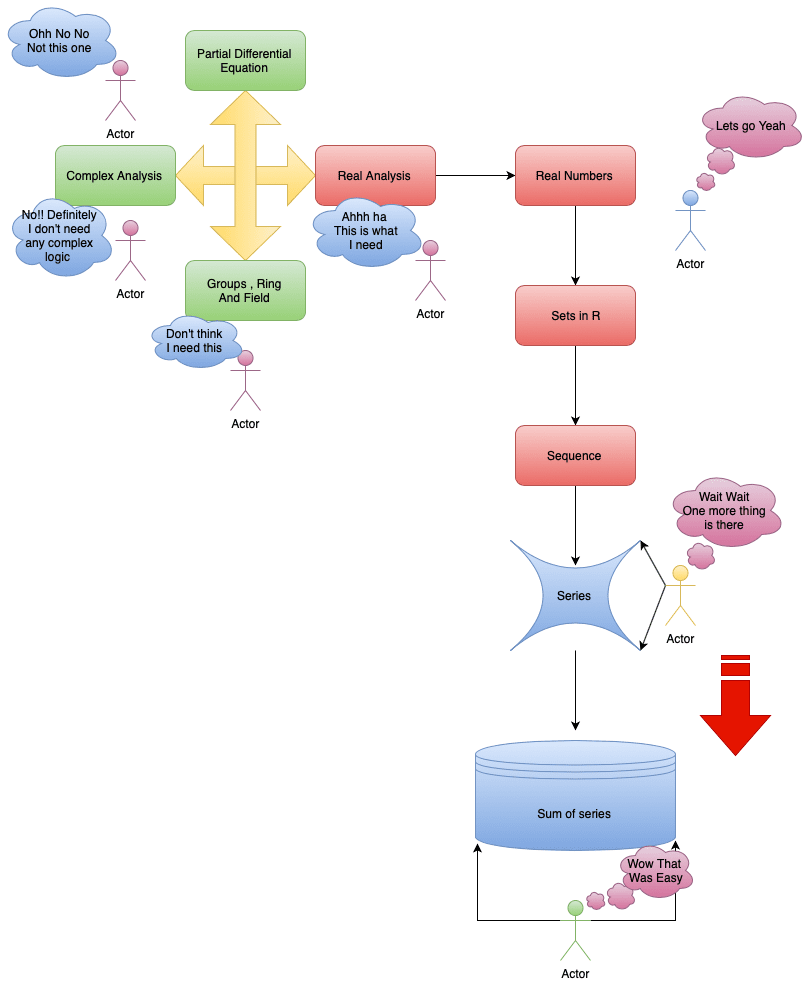# Let’s have a look into the graphs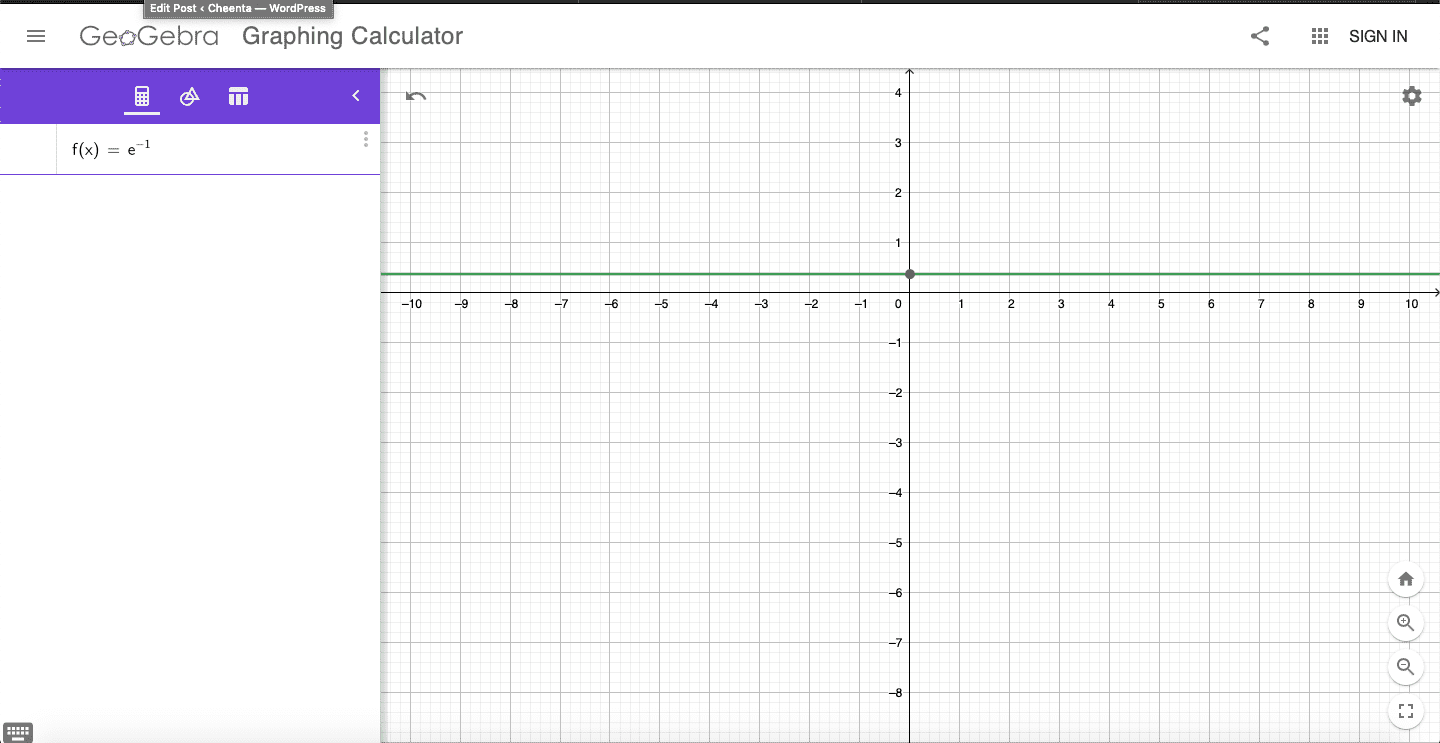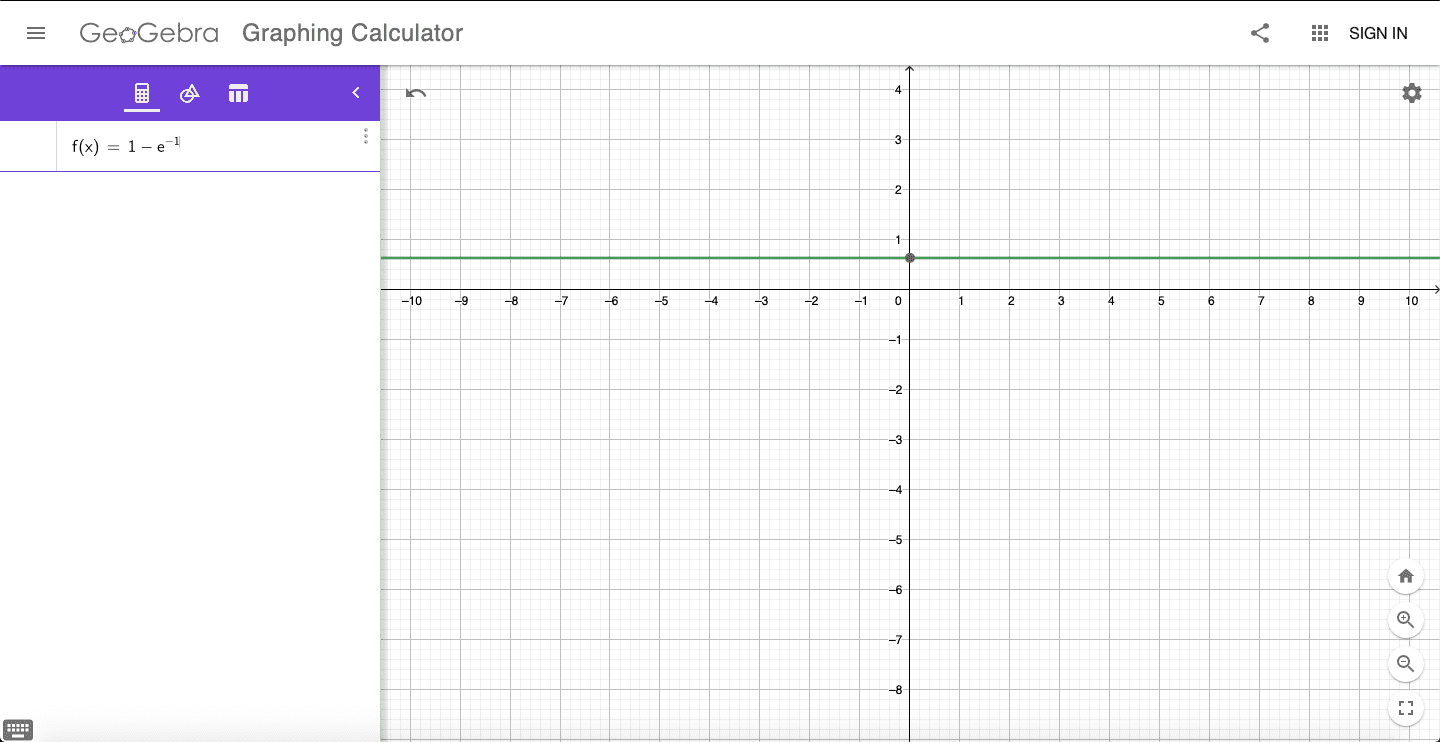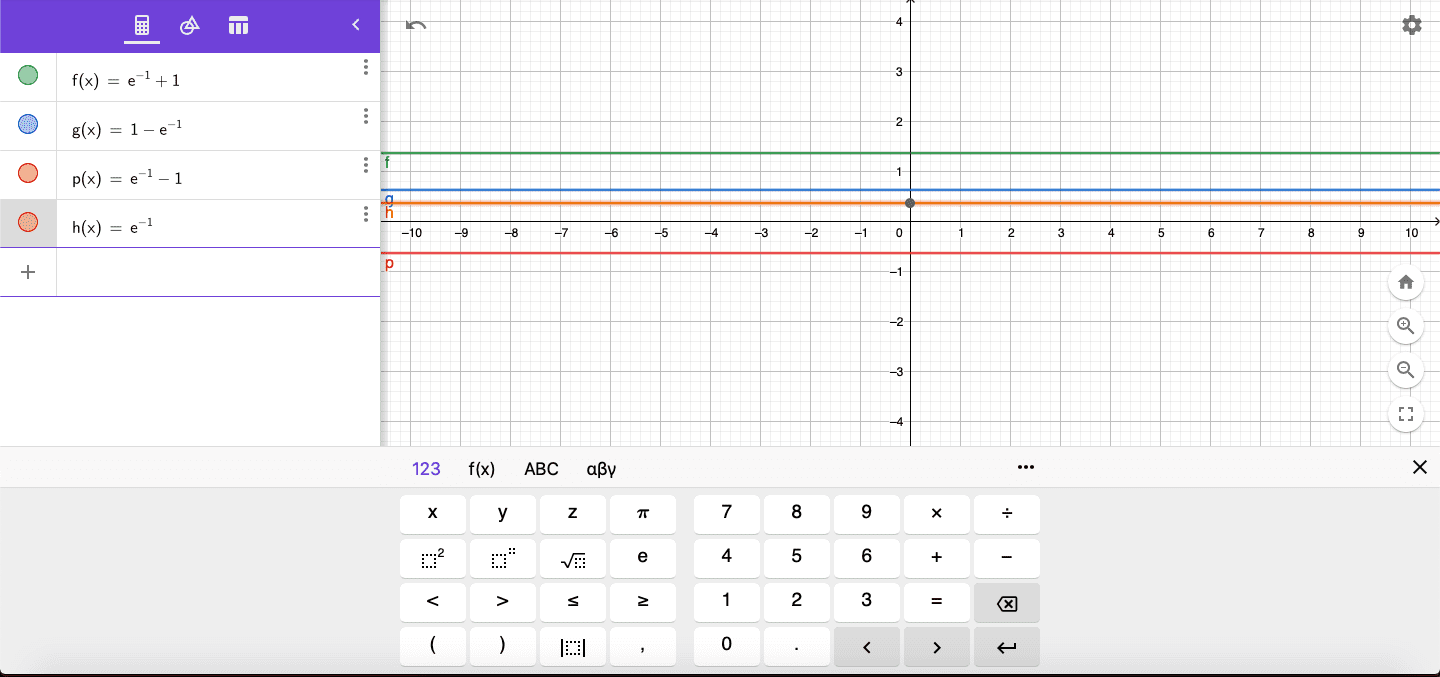# Do You Know ????# Connected Program at Cheenta

#### College Mathematics Program

The higher mathematics program caters to advanced college and university students. It is useful for I.S.I. M.Math Entrance, GRE Math Subject Test, TIFR Ph.D. Entrance, I.I.T. JAM. The program is problem driven. We work with candidates who have a deep love for mathematics. This program is also useful for adults continuing who wish to rediscover the world of mathematics.

# Similar Problems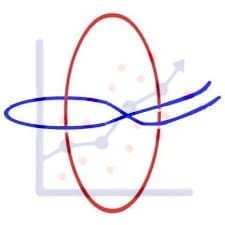## ISI MStat PSB 2006 Problem 8 | Bernoullian Beauty

This is a very simple and regular sample problem from ISI MStat PSB 2009 Problem 8. It It is based on testing the nature of the mean of Exponential distribution. Give it a Try it !## ISI MStat PSB 2009 Problem 8 | How big is the Mean?

This is a very simple and regular sample problem from ISI MStat PSB 2009 Problem 8. It It is based on testing the nature of the mean of Exponential distribution. Give it a Try it !## ISI MStat PSB 2009 Problem 4 | Polarized to Normal

This is a very beautiful sample problem from ISI MStat PSB 2009 Problem 4. It is based on the idea of Polar Transformations, but need a good deal of observation o realize that. Give it a Try it !## ISI MStat PSB 2009 Problem 6 | abNormal MLE of Normal

This is a very beautiful sample problem from ISI MStat PSB 2009 Problem 6. It is based on the idea of Restricted Maximum Likelihood Estimators, and Mean Squared Errors. Give it a Try it !## ISI MStat PSB 2009 Problem 3 | Gamma is not abNormal

This is a very simple but beautiful sample problem from ISI MStat PSB 2009 Problem 3. It is based on recognizing density function and then using CLT. Try it !## ISI MStat PSB 2009 Problem 1 | Nilpotent Matrices

This is a very simple sample problem from ISI MStat PSB 2009 Problem 1. It is based on basic properties of Nilpotent Matrices and Skew-symmetric Matrices. Try it !## ISI MStat PSB 2006 Problem 2 | Cauchy & Schwarz come to rescue

This is a very subtle sample problem from ISI MStat PSB 2006 Problem 2. After seeing this problem, one may think of using Lagrange Multipliers, but one can just find easier and beautiful way, if one is really keen to find one. Can you!

## Problem on Inequality | ISI – MSQMS – B, 2018 | Problem 2a

Try this problem from ISI MSQMS 2018 which involves the concept of Inequality. You can use the sequential hints provided to solve the problem.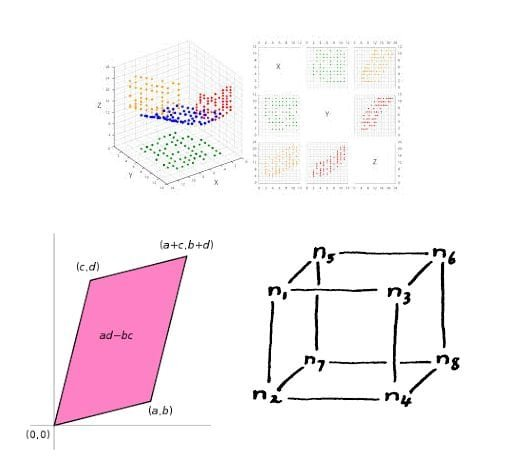## Data, Determinant and Simplex

This problem is a beautiful problem connecting linear algebra, geometry and data. Go ahead and dwelve into the glorious connection.

## Problem on Integral Inequality | ISI – MSQMS – B, 2015

Try this problem from ISI MSQMS 2015 which involves the concept of Integral Inequality and real analysis. You can use the sequential hints provided to solve the problem.
Categories

# What are we learning?

Sequences & Subsequences are the key features in the filed of real analysis. We will see how to imply these concepts in our problem

[h5p id="10"]

# Understand the problem

Let $s_n$ = 1+$\frac{1}{1!}$+$\frac{1}{2!}$+……..+$\frac{1}{n!}$ for n $\in$ $\mathbb{N}$ Then which of the following is TRUE for the sequence {$s_{n}\}^\infty_{n=1}$:   (a) {$s_{n}\}^\infty_{n=1}$ converges in $\mathbb{Q}$.   (b) {$s_{n}\}^\infty_{n=1}$ is a Cauchy sequence but does not converges to $\mathbb{Q}$.   (c) The subsequence  {$s_{k^n}\}^\infty_{n=1}$ is convergent in $\mathbb{R}$ when k is a even natural number.   (d) {$s_{n}\}^\infty_{n=1}$ is not a Cauchy sequence.
IIT Jam 2018
Easy
##### Suggested Book
 Calculus: Multi-Variable Calculus and Linear Algebra with Applications to Differential Equations and Probability – Vol 2 Tom M. Apostol

Do you really need a hint? Try it first!

I am going to give you 3 clues in the beginning you try to work out using them. Then I will elaborate this clues in the following hints  (I) Every convergent sequence is a Cauchy sequence  (II)Every subsequence of a convergent sequence is convergent  (III)Consider then term 1+$\frac{1}{1!}$+$\frac{1}{2!}$+……..+$\frac{1}{n!}$ Does this remind you any well known series?

I wil start with (III) consider $e^x$=1+$\frac{x}{1!}$+$\frac{x^2}{2!}$+……..+$\frac{x^n}{n!}$ Isn’t the seris that we have to , is the value at x=1. Hence the given series$\rightarrow$ e $\in$ $\mathbb{R}$ \ $\mathbb{Q}$

So option (a) is incorrect.

Every subsequence of a convergent sequence is convergent so {$s_{k^n}\}^\infty_{n=1}$ is convergent not only for even k, but for any $k \in \Bbb N$. So option (c) is incorrect.

Every convergent sequence is a Cauchy sequence so option (d) is incorrect and $e \in$ $\mathbb{R}$ so the given subsequence is convergent in $\mathbb{R}$. So only option (b) is correct.

# Look at the knowledge graph…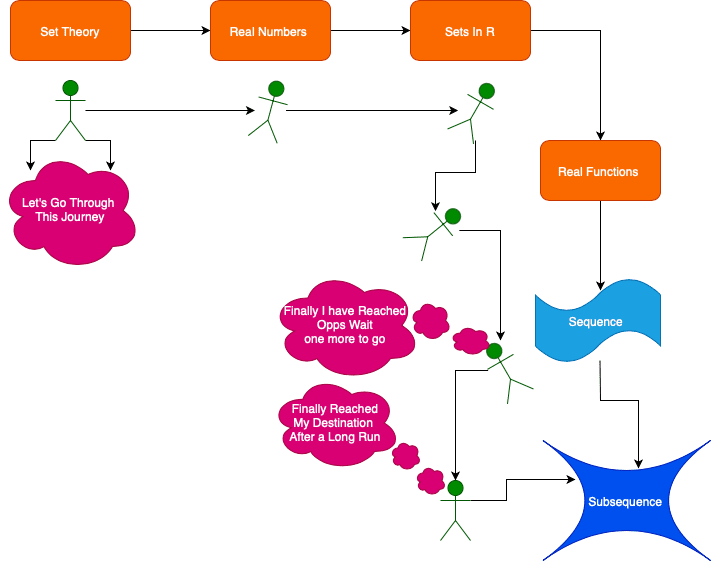# Play with graph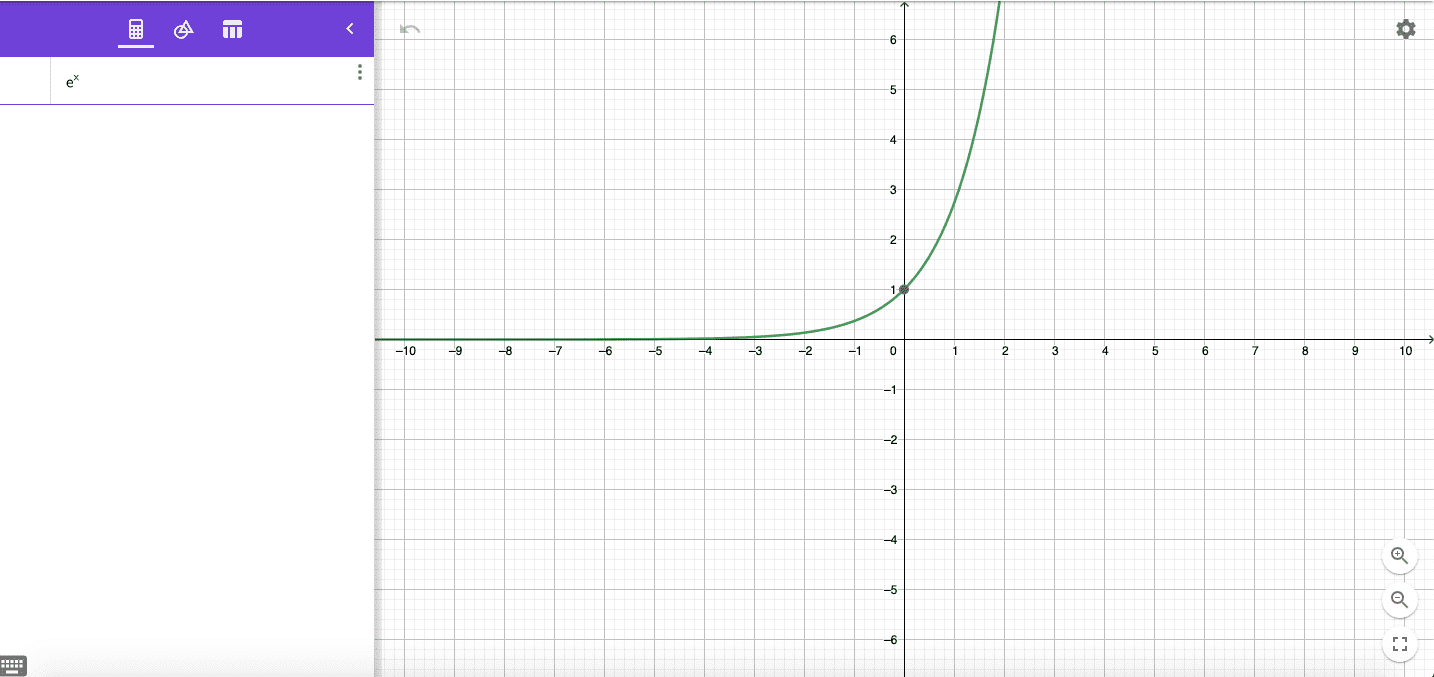# Fun fact: Do you know that this man has a sequence named after him?# Connected Program at Cheenta

#### College Mathematics Program

The higher mathematics program caters to advanced college and university students. It is useful for I.S.I. M.Math Entrance, GRE Math Subject Test, TIFR Ph.D. Entrance, I.I.T. JAM. The program is problem driven. We work with candidates who have a deep love for mathematics. This program is also useful for adults continuing who wish to rediscover the world of mathematics.

# Similar Problems## ISI MStat PSB 2006 Problem 8 | Bernoullian Beauty

This is a very simple and regular sample problem from ISI MStat PSB 2009 Problem 8. It It is based on testing the nature of the mean of Exponential distribution. Give it a Try it !## ISI MStat PSB 2009 Problem 8 | How big is the Mean?

This is a very simple and regular sample problem from ISI MStat PSB 2009 Problem 8. It It is based on testing the nature of the mean of Exponential distribution. Give it a Try it !## ISI MStat PSB 2009 Problem 4 | Polarized to Normal

This is a very beautiful sample problem from ISI MStat PSB 2009 Problem 4. It is based on the idea of Polar Transformations, but need a good deal of observation o realize that. Give it a Try it !## ISI MStat PSB 2009 Problem 6 | abNormal MLE of Normal

This is a very beautiful sample problem from ISI MStat PSB 2009 Problem 6. It is based on the idea of Restricted Maximum Likelihood Estimators, and Mean Squared Errors. Give it a Try it !## ISI MStat PSB 2009 Problem 3 | Gamma is not abNormal

This is a very simple but beautiful sample problem from ISI MStat PSB 2009 Problem 3. It is based on recognizing density function and then using CLT. Try it !## ISI MStat PSB 2009 Problem 1 | Nilpotent Matrices

This is a very simple sample problem from ISI MStat PSB 2009 Problem 1. It is based on basic properties of Nilpotent Matrices and Skew-symmetric Matrices. Try it !## ISI MStat PSB 2006 Problem 2 | Cauchy & Schwarz come to rescue

This is a very subtle sample problem from ISI MStat PSB 2006 Problem 2. After seeing this problem, one may think of using Lagrange Multipliers, but one can just find easier and beautiful way, if one is really keen to find one. Can you!

## Problem on Inequality | ISI – MSQMS – B, 2018 | Problem 2a

Try this problem from ISI MSQMS 2018 which involves the concept of Inequality. You can use the sequential hints provided to solve the problem.## Data, Determinant and Simplex

This problem is a beautiful problem connecting linear algebra, geometry and data. Go ahead and dwelve into the glorious connection.

## Problem on Integral Inequality | ISI – MSQMS – B, 2015

Try this problem from ISI MSQMS 2015 which involves the concept of Integral Inequality and real analysis. You can use the sequential hints provided to solve the problem.